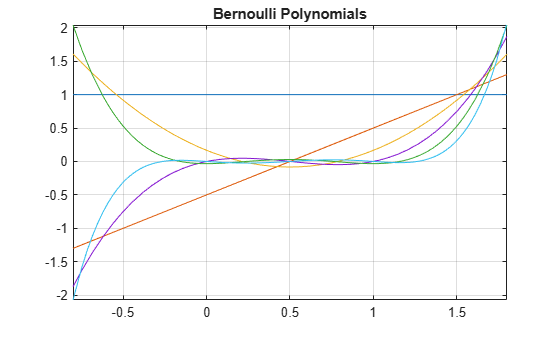# bernoulli

Bernoulli numbers and polynomials

## Syntax

``bernoulli(n)``
``bernoulli(n,x)``

## Description

example

````bernoulli(n)` returns the `n`th Bernoulli number.```

example

````bernoulli(n,x)` returns the `n`th Bernoulli polynomial.```

## Examples

### Bernoulli Numbers with Odd and Even Indices

The 0th Bernoulli number is `1`. The next Bernoulli number can be `-1/2` or `1/2`, depending on the definition. The `bernoulli` function uses `-1/2`. The Bernoulli numbers with even indices ```n > 1``` alternate the signs. Any Bernoulli number with an odd index `n > 2` is `0`.

Compute the even-indexed Bernoulli numbers with the indices from `0` to `10`. Because these indices are not symbolic objects, `bernoulli` returns floating-point results.

`bernoulli(0:2:10)`
```ans = 1.0000 0.1667 -0.0333 0.0238 -0.0333 0.0758```

Compute the same Bernoulli numbers for the indices converted to symbolic objects:

`bernoulli(sym(0:2:10))`
```ans = [ 1, 1/6, -1/30, 1/42, -1/30, 5/66]```

Compute the odd-indexed Bernoulli numbers with the indices from `1` to `11`:

`bernoulli(sym(1:2:11))`
```ans = [ -1/2, 0, 0, 0, 0, 0]```

### Bernoulli Polynomials

For the Bernoulli polynomials, use `bernoulli` with two input arguments.

Compute the first, second, and third Bernoulli polynomials in variables `x`, `y`, and `z`, respectively:

```syms x y z bernoulli(1, x) bernoulli(2, y) bernoulli(3, z)```
```ans = x - 1/2 ans = y^2 - y + 1/6 ans = z^3 - (3*z^2)/2 + z/2```

If the second argument is a number, `bernoulli` evaluates the polynomial at that number. Here, the result is a floating-point number because the input arguments are not symbolic numbers:

`bernoulli(2, 1/3)`
```ans = -0.0556```

To get the exact symbolic result, convert at least one of the numbers to a symbolic object:

`bernoulli(2, sym(1/3))`
```ans = -1/18```

### Plot Bernoulli Polynomials

Plot the first six Bernoulli polynomials.

```syms x fplot(bernoulli(0:5, x), [-0.8 1.8]) title('Bernoulli Polynomials') grid on```### Handle Expressions Containing Bernoulli Polynomials

Many functions, such as `diff` and `expand`, handles expressions containing `bernoulli`.

Find the first and second derivatives of the Bernoulli polynomial:

```syms n x diff(bernoulli(n,x^2), x)```
```ans = 2*n*x*bernoulli(n - 1, x^2)```
`diff(bernoulli(n,x^2), x, x)`
```ans = 2*n*bernoulli(n - 1, x^2) +... 4*n*x^2*bernoulli(n - 2, x^2)*(n - 1)```

Expand these expressions containing the Bernoulli polynomials:

`expand(bernoulli(n, x + 3))`
```ans = bernoulli(n, x) + (n*(x + 1)^n)/(x + 1) +... (n*(x + 2)^n)/(x + 2) + (n*x^n)/x```
`expand(bernoulli(n, 3*x))`
```ans = (3^n*bernoulli(n, x))/3 + (3^n*bernoulli(n, x + 1/3))/3 +... (3^n*bernoulli(n, x + 2/3))/3```

## Input Arguments

collapse all

Index of the Bernoulli number or polynomial, specified as a nonnegative integer, symbolic nonnegative integer, variable, expression, function, vector, or matrix. If `n` is a vector or matrix, `bernoulli` returns Bernoulli numbers or polynomials for each element of `n`. If one input argument is a scalar and the other one is a vector or a matrix, `bernoulli(n,x)` expands the scalar into a vector or matrix of the same size as the other argument with all elements equal to that scalar.

Polynomial variable, specified as a symbolic variable, expression, function, vector, or matrix. If `x` is a vector or matrix, `bernoulli` returns Bernoulli numbers or polynomials for each element of `x`. When you use the `bernoulli` function to find Bernoulli polynomials, at least one argument must be a scalar or both arguments must be vectors or matrices of the same size. If one input argument is a scalar and the other one is a vector or a matrix, `bernoulli(n,x)` expands the scalar into a vector or matrix of the same size as the other argument with all elements equal to that scalar.

collapse all

### Bernoulli Polynomials

The Bernoulli polynomials are defined as follows:

`$\frac{t{e}^{xt}}{{e}^{t}-1}=\sum _{n=0}^{\infty }\mathrm{bernoulli}\left(n,x\right)\frac{{t}^{n}}{n!}\text{\hspace{0.17em}}$`

### Bernoulli Numbers

The Bernoulli numbers are defined as follows:

`$\mathrm{bernoulli}\left(n\right)=\mathrm{bernoulli}\left(n,0\right)$`